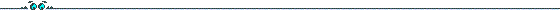## Preprints and Thesis of Tanja LangeFor a description of hyperellitptic curves and Koblitz curves in special visit KoblitzC.html.

• A detailed preprint "Efficient Arithmetic on Hyperelliptic Koblitz Curves" (83 pages) dealing with arbitrary genus and characteristic. It appeared as Preprint 2, 2001, Institute for Experimental Mathematics.
• A short version containing only the results is here. It appeared June 2,2001 under ISBN 90-74249-25-6.
• My thesis "Fast Arithmetic on Hyperelliptic Curves" is out now. The file contains most of the preprint plus some additional stuff on explicit formula and trace-zero varieties. It appeared as Preprint 4, 2002, Institute for Experimental Mathematics. It appeared as Preprint 4, 2002, Institute for Experimental Mathematics.
• The preprint "Efficient Arithmetic on Genus 2 Hyperelliptic Curves over Finite Fields via Explicit Formulae" is concerned with Genus 2 curves over arbitrary finite fields and the most efficient arithmetic on them obtained via explicit formulae. See as well Cryptology ePrint Archive, 2002/121.
The files below give the updated version as of December 9, 2002. The first version was August 16, 2002.
• In the preprint "Inversion-Free Arithmetic on Genus 2 Hyperelliptic Curves" we adopt the term 'projective coordinates' used for elliptic curves to denote a representation that is not normalized. The advantage is that one does not use inversions with this representation, on the other hand more mulitplications are needed. For hardware the choice of characteristic two is especially interesting and using these formulae it is faster than odd characteristic. For Koblitz-Curves (see above) these formulae are fastest. This is the second version of December 9, 2002 (see also Cryptology ePrint Archive, 2002/147).
• The preprint "Weighted coordinates on Genus 2 Hyperelliptic Curves" is the third in a line dealing with arithmetic on hyperelliptic curves. Like in the previous paper we avoid inversions and show how to obtain faster formulae for doublings. Furthermore we are concerned with scalar multiplications and look for optimal matches of systems of coordinates to compute them -- this depends on the system of the input and also on the number of precomputations to be stored. In this paper the cases of odd and even characteristic are treated separately and as the tables show this also implies a small improvement over the previous paper. This is the first version of October,11, 2002 (see also Cryptology ePrint Archive, 2002/153).
• The paper "Formulae for Arithmetic on Genus 2 Hyperelliptic Curves" gives a thourough comparison of arithmetic on hyperelliptic curves of genus 2. This is supposed to be the journal version, partially containing the material of the previous three papers adding some new features. This is the accepted version of May, 25, 2004.
• In the paper "Trace Zero Subvariety for Cryptosystems" we present a kind of group suitable for cryptographic applications: the trace zero subvariety. The construction is based on Weil descent from curves of genus two over extension fields \$\F_{p^n}\$, \$n=3\$. On the Jacobian of the curve the group can be seen as a prime order subgroup, however, considering the construction as Weil descent we can argue that the security is equivalent to that of groups based on low-genus hyperelliptic curves over prime fields. The advantage is that the complexity to compute scalar multiples is lower, as one can make use of the Frobenius endomorphism of the initial curve. Thus the trace zero subvariety can be used efficiently in protocols based on the discrete logarithm problem. This is the version of February, 24, 2004 (see also Cryptology ePrint Archive, 2003/094).
• The preprint Mathematical Background of Public Key Cryptography (joint work with G. Frey) gives an overview on public key cryptography. The paper is aimed towards an audience with mathematic background who want to learn about applications in cryptography but we also try to reach the non-expert providing a lot of references to recent publications. It appeared as Preprint 10 (2003) in the preprint series of the Institute for Experimental Mathematics.

to Tanja Lange's Homepage
to Tanja Lange's Koblitz curves page
zu Tanja Langes Homepage (deutsch)